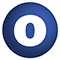ap

# PresburgerTools

### Related Doc: package ap

#### object PresburgerTools

A collection of tools for analysing and transforming formulae in Presburger arithmetic

Linear Supertypes
AnyRef, Any
Ordering
1. Alphabetic
2. By inheritance
Inherited
1. PresburgerTools
2. AnyRef
3. Any
1. Hide All
2. Show all
Visibility
1. Public
2. All

### Value Members

1. #### final def !=(arg0: Any): Boolean

Definition Classes
AnyRef → Any
2. #### final def ##(): Int

Definition Classes
AnyRef → Any
3. #### final def ==(arg0: Any): Boolean

Definition Classes
AnyRef → Any
4. #### final def asInstanceOf[T0]: T0

Definition Classes
Any
5. #### def bounds(objective: LinearCombination, constraint: Conjunction): Option[(IdealInt, IdealInt)]

Check whether the function `objective` has both a lower and an upper bound subject to `constraint`, and return such bounds.

Check whether the function `objective` has both a lower and an upper bound subject to `constraint`, and return such bounds.

6. #### def clone(): AnyRef

Attributes
protected[java.lang]
Definition Classes
AnyRef
Annotations
@throws( ... )

9. #### def distinctInterpretation[T <: Term](terms: Set[T], order: TermOrder): Map[ConstantTerm, IdealInt]

Find an interpretation of the constants in the given terms that will make all terms evaluate to pairwise distinct integers.

10. #### def elimQuantifiersWithPreds(c: Conjunction): Conjunction

Quantifier elimination procedure that can also handle uninterpreted predicates, provided that predicates never occur in the scope of quantifiers.

Quantifier elimination procedure that can also handle uninterpreted predicates, provided that predicates never occur in the scope of quantifiers. Quantifiers above predicate occurrences are left in the formula. The method can also handle formulas with bit-vector arithmetic or non-linear multiplication, and is optimised for post-processing of interpolants.

11. #### def eliminatePredicates(c: Conjunction, axioms: Conjunction, order: TermOrder): Conjunction

Compute the most general quantifier-free formula without uninterpreted predicates that implies the given formula, modulo the given axioms.

Compute the most general quantifier-free formula without uninterpreted predicates that implies the given formula, modulo the given axioms. If the input formula or the axioms contain quantifiers, this might not terminate.

12. #### def enumDisjuncts(disjunction: Conjunction): Iterator[Conjunction]

Enumerate the disjuncts of a formula that already is in DNF.

13. #### def enumModels(formula: Conjunction): Iterator[Conjunction]

Enumerate the models of a given formula.

Enumerate the models of a given formula. Currently, we assume that the formula does not contain predicates and only existential quantifiers (this could be relaxed)

14. #### final def eq(arg0: AnyRef): Boolean

Definition Classes
AnyRef
15. #### def equals(arg0: Any): Boolean

Definition Classes
AnyRef → Any
16. #### def finalize(): Unit

Attributes
protected[java.lang]
Definition Classes
AnyRef
Annotations
@throws( classOf[java.lang.Throwable] )
17. #### final def getClass(): Class[_]

Definition Classes
AnyRef → Any

19. #### def hashCode(): Int

Definition Classes
AnyRef → Any

21. #### final def isInstanceOf[T0]: Boolean

Definition Classes
Any

27. #### def lowerBound(objective: LinearCombination, constraint: Conjunction): Option[IdealInt]

Check whether the function `objective` has a lower bound subject to `constraint`, and return such a bound.

Check whether the function `objective` has a lower bound subject to `constraint`, and return such a bound.

29. #### def minimiseFormula(c: Conjunction): Conjunction

Minimise the given formula by eliminating unnecessary disjuncts.

Minimise the given formula by eliminating unnecessary disjuncts. This is a stronger version of the simplification performed by `ReduceWithConjunction`, and also simplifies formulae `a & (b | c) & (b | c')` where `c & c'` is unsatisfiable.

30. #### final def ne(arg0: AnyRef): Boolean

Definition Classes
AnyRef
31. #### def nonDNFEnumDisjuncts(formula: Conjunction): Iterator[Conjunction]

Enumerate the disjuncts of a formula (which might not be in DNF).

32. #### final def notify(): Unit

Definition Classes
AnyRef
33. #### final def notifyAll(): Unit

Definition Classes
AnyRef
34. #### final def synchronized[T0](arg0: ⇒ T0): T0

Definition Classes
AnyRef
35. #### def toDNF(formula: Conjunction): Conjunction

Turn a formula into DNF.

Turn a formula into DNF. The DNF might not be complete in the sense that a formula ` a & b | a & c ` might only be normalised to ` a & (b | c) `

36. #### def toPrenex(c: Conjunction): Conjunction

Convert a given formula to prenex form.

Convert a given formula to prenex form. TODO: do something special for divisibilities?

37. #### def toString(): String

Definition Classes
AnyRef → Any
38. #### final def wait(): Unit

Definition Classes
AnyRef
Annotations
@throws( ... )
39. #### final def wait(arg0: Long, arg1: Int): Unit

Definition Classes
AnyRef
Annotations
@throws( ... )
40. #### final def wait(arg0: Long): Unit

Definition Classes
AnyRef
Annotations
@throws( ... )